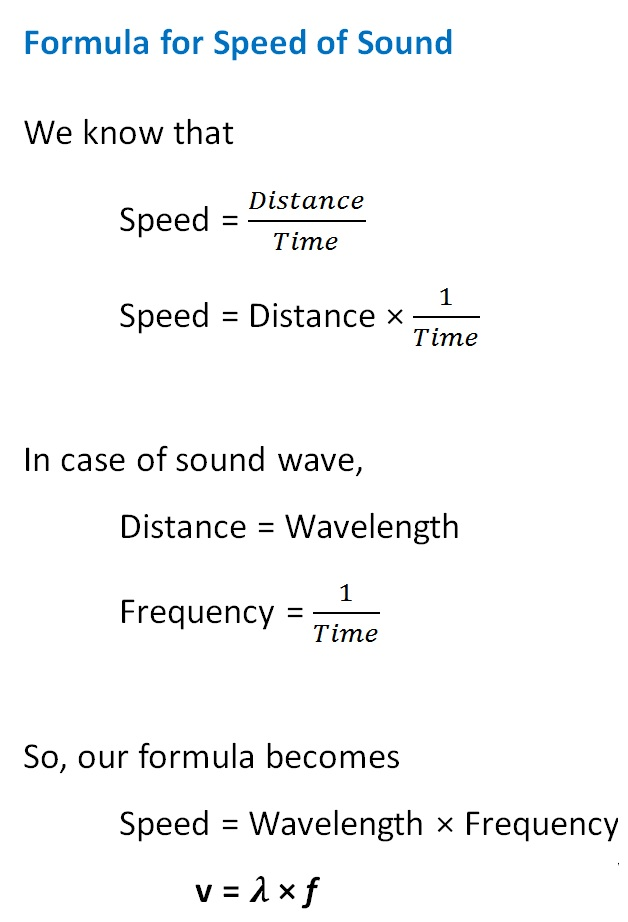Concepts

Class 9
Chapter 12 Class 9 - Sound

## What is speed of Sound?

It is 344 meter per second at 22 degree celsius in air

## Is speed of sound constant?

No

It changes according to

• Medium it travels (Solid, Liquid, Gas)
• Temperature
• Humidity of air

## How does speed of sound depend upon medium

We know that sound requires a medium to travel (like solid, liquid, gas)

It travels fastest in solids, slower in liquids and slowest in air (gas)

Example

 Medium Speed of Sound Air 346 m/s Water 1531 m/s iron 5950 m/s

## How does speed of sound depend upon temperature?

If we increase temperature,speed of sound increases

If we decrease the temperature, speed of sound decreases

 Air Temperature Speed of Sound 0 degree 331 m/s 15 degree 340 m/s 20 degree 343 m/s 25 degree 346 m/s

## How does speed of sound depend upon humidity

Humidity is the amount of moisture in the air

More the humidity, more is the speed of sound

Sound travels faster at 100% humidity (very moist air) as compared to 0% humidity (completely dry air)

## How to Measure Speed of Sound

Speed of Sound Wave = Wavelength*Frequency

Explanation

We know that## Questions

Question 1 Page 167 - In which of the three media, air, water or iron, does sound travel the fastest at a particular temperature?

Question 2 Page 166 (Right Top) - How are the wavelength and frequency of a sound wave related to its speed?

Question 3 Page 166 (Right Top) - Calculate the wavelength of a sound wave whose frequency is 220 Hz and speed is 440 m/s in a given medium.

Question 4 Page 166 (Right Top) - A person is listening to a tone of 500 Hz sitting at a distance of 450 m from the source of the sound. What is the time interval between successive compressions from the source?

NCERT Question 6 - Flash and thunder are produced simultaneously. But thunder is heard a few seconds after the flash is seen, why?

NCERT Question 7 - A person has a hearing range from 20 Hz to 20 kHz. What are the typical wavelengths of sound waves in air corresponding to these two frequencies? Take the speed of sound in air as 344 m s –1 .

NCERT Question 8 - Two children are at opposite ends of an aluminium rod. One strikes the end of the rod with a stone. Find the ratio of times taken by the sound wave in air and in aluminium to reach the second child.

NCERT Question 9 - The frequency of a source of sound is 100 Hz. How many times does it vibrate in a minute?

NCERT Question 13 - A stone is dropped from the top of a tower 500 m high into a pond of water at the base of the tower. When is the splash heard at the top? Given, g = 10 m s –2 . and speed of sound = 340 m s –1 .

Learn in your speed, with individual attention - Teachoo Maths 1-on-1 Class